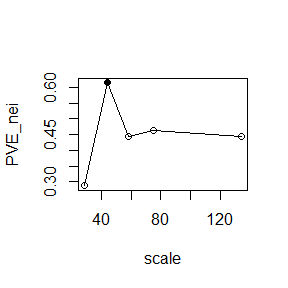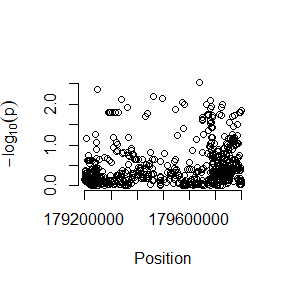# rNeighborGWAS

## Overview

This R package provides a set of functions to test neighbor effects in marker-based regressions. In this vignette, we first estimate an effective range of neighbor effects, and then perform an association mapping of neighbor effects. See Sato et al. (2019) for model description.

## Input files

First, let us see the data structure of input files necessary for the neighbor GWAS. Here is an example using a phenotype simulated from “TTN” genotype in the “gaston” package (Perdry & Dandine-Roulland 2020). Genotype data are a matrix including individuals (rows) x markers (columns) with -1 or +1 digit for bialleles. A spatial map indicates a individual distribution along x- and y-axes in a 2D space.

set.seed(1234)
library(rNeighborGWAS)

# convert "TTN" genotype data into a rNeighborGWAS format
data("TTN", package="gaston")
x <- gaston::as.bed.matrix(TTN.gen, TTN.fam, TTN.bim)
g <- gaston2neiGWAS(x)

# simulate "fake_nei" dataset using nei_simu()
geno <- g$geno gmap <- g$gmap
x <- runif(nrow(geno),1,100)
y <- runif(nrow(geno),1,100)
smap <- cbind(x,y)
grouping <- c(rep(1,nrow(geno)/2), rep(2,nrow(geno)/2), 2)
pheno <- nei_simu(geno=geno, smap=smap, scale=43,
grouping=grouping, n_causal=50,
pveB=0.3, pve=0.6
)

fake_nei <- list()
fake_nei[] <- geno
fake_nei[] <- gmap
fake_nei[] <- smap
fake_nei[] <- data.frame(pheno,grouping)
names(fake_nei) <- c("geno","gmap","smap","pheno")

fake_nei$geno[1:5,1:10] # Note: 0 indicates heterozygotes #> rs7571247 rs3813253 rs6760059 rs16866263 rs77946091 rs77711640 #> HG00096 1 1 1 1 1 1 #> HG00097 1 1 1 1 1 1 #> HG00099 0 1 1 1 1 1 #> HG00100 1 -1 -1 1 1 1 #> HG00101 1 1 1 1 1 1 #> rs12618595 rs75964992 rs7585689 rs75075682 #> HG00096 1 1 1 1 #> HG00097 1 1 1 1 #> HG00099 1 1 1 1 #> HG00100 1 1 1 1 #> HG00101 1 1 1 1 head(fake_nei$smap)
#>             x        y
#> [1,] 61.31820 84.74344
#> [2,] 62.71456 74.89343
#> [3,] 86.23062 83.12688
#> [4,] 64.39075 13.66211
#> [5,]  1.94008 79.61674
#> [6,] 24.02250 36.19509

## Variation partitioning across a space

To estimate an effective range of neighbor effects, we calculate a heritability-like metric, that is the proportion of phenotypic variation explained by neighbor effects (PVE_nei). The optimal scale of neighbor effects is analyzed by how PVE_nei approaches to a plateau.

scale_seq <- quantile(dist(fake_nei$smap),c(0.2*rep(1:5))) pve_out <- calc_PVEnei(geno=fake_nei$geno, pheno=fake_nei$pheno[,1], smap=fake_nei$smap, scale_seq=scale_seq,
addcovar=as.matrix(fake_nei$pheno$grouping),
grouping=fake_nei$pheno$grouping
)
#> scale = 28.937
#> scale = 43.89
#> scale = 58.376
#> scale = 74.836
#> scale = 134.43
delta_PVE(pve_out)#>      scale     PVEnei
#> 43.8900867  0.6146725

## Association mapping

Based on the estimated scale of neighbor effects, we then perform genome-wide association mapping of neighbor effects as follows.

scale <- 43.9
gwas_out <- neiGWAS(geno=fake_nei$geno, pheno=fake_nei$pheno[,1],
gmap=fake_nei$gmap, smap=fake_nei$smap,
scale=scale, addcovar=as.matrix(fake_nei$pheno$grouping),
grouping=fake_nei$pheno$grouping
)

gaston::manhattan(gwas_out)pheno=fake_nei$pheno[,1], addcovar=as.matrix(fake_nei$pheno$grouping) ) ## Binary phenotype The line of analyses can work with logistic mixed models that allow a binary phenotype. Convert the phenotype values into 0 or 1, and choose “binary” in the “response” argument. fake_nei$pheno[,1][fake_nei$pheno[,1]>mean(fake_nei$pheno[,1])] <- 1
fake_nei$pheno[,1][fake_nei$pheno[,1]!=1] <- 0

pve_out <- calc_PVEnei(geno=fake_nei$geno, pheno=fake_nei$pheno[,1],
smap=fake_nei$smap, scale_seq=scale_seq, addcovar=as.matrix(fake_nei$pheno$grouping), grouping=fake_nei$pheno$grouping, response="binary" ) gwas_out <- neiGWAS(geno=fake_nei$geno, pheno=fake_nei$pheno[,1], gmap=fake_nei$gmap, smap=fake_nei$smap, scale=scale, addcovar=as.matrix(fake_nei$pheno$grouping), grouping=fake_nei$pheno$grouping, response="binary" ) gaston::manhattan(gwas_out) gaston::qqplot.pvalues(gwas_out$p)

gwas_out <- nei_lmm(geno=fake_nei$geno, g_nei=g_nei, pheno=fake_nei$pheno[,1],
addcovar=as.matrix(fake_nei$pheno$grouping),
response="binary"
)